Open in App
Not now

# Understanding Data Attribute Types | Qualitative and Quantitative

• Difficulty Level : Easy
• Last Updated : 08 Jul, 2022

Prerequisites: Data Mining

When we talk about data mining, we usually discuss knowledge discovery from data. To get to know about the data it is necessary to discuss data objects, data attributes, and types of data attributes. Mining data includes knowing about data, finding relations between data. And for this, we need to discuss data objects and attributes.

Data objects are the essential part of a database. A data object represents the entity. Data Objects are like a group of attributes of an entity. For example, a sales data object may represent customers, sales, or purchases. When a data object is listed in a database they are called data tuples.

Attribute:
It can be seen as a data field that represents the characteristics or features of a data object. For a customer, object attributes can be customer Id, address, etc. We can say that a set of attributes used to describe a given object are known as attribute vector or feature vector.

### Type of attributes :

This is the First step of Data-preprocessing. We differentiate between different types of attributes and then preprocess the data. So here is the description of attribute types.

1. Qualitative (Nominal (N), Ordinal (O), Binary(B)).
2.  Quantitative (Numeric, Discrete, Continuous)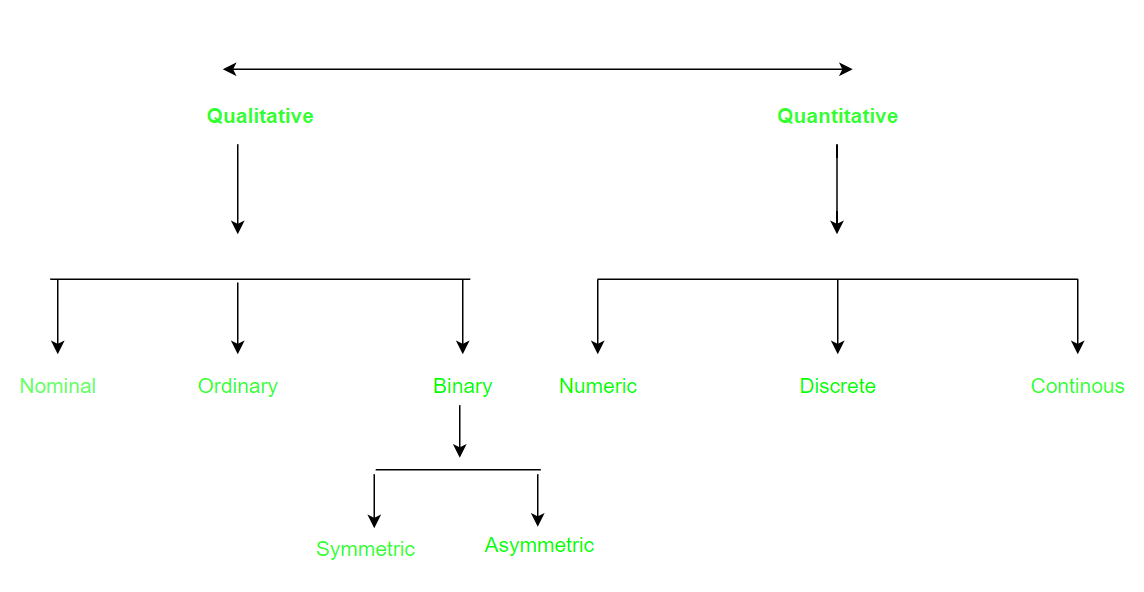### Qualitative Attributes:

1. Nominal Attributes – related to names: The values of a Nominal attribute are names of things, some kind of symbols. Values of Nominal attributes represents some category or state and that’s why nominal attribute also referred as categorical attributes and there is no order (rank, position) among values of the nominal attribute.
Example :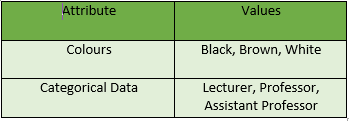2. Binary Attributes: Binary data has only 2 values/states. For Example yes or no, affected or unaffected, true or false.

• Symmetric: Both values are equally important (Gender).
• Asymmetric: Both values are not equally important (Result).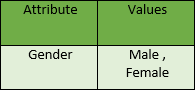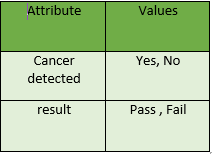3. Ordinal Attributes : The Ordinal Attributes contains values that have a meaningful sequence or ranking(order) between them, but the magnitude between values is not actually known, the order of values that shows what is important but don’t indicate how important it is.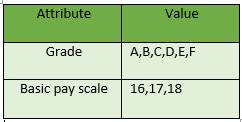### Quantitative Attributes:

1. Numeric: A numeric attribute is quantitative because, it is a measurable quantity, represented in integer or real values. Numerical attributes are of 2 types, interval, and ratio

• An interval-scaled attribute has values, whose differences are interpretable, but the numerical attributes do not have the correct reference point, or we can call zero points. Data can be added and subtracted at an interval scale but can not be multiplied or divided. Consider an example of temperature in degrees Centigrade. If a day’s temperature of one day is twice of the other day we cannot say that one day is twice as hot as another day.
• A ratio-scaled attribute is a numeric attribute with a fix zero-point. If a measurement is ratio-scaled, we can say of a value as being a multiple (or ratio) of another value. The values are ordered, and we can also compute the difference between values, and the mean, median, mode, Quantile-range, and Five number summary can be given.

2. Discrete : Discrete data have finite values it can be numerical and can also be in categorical form. These attributes has finite or countably infinite set of values.

Example: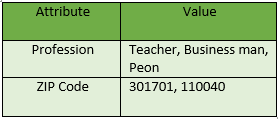3. Continuous: Continuous data have an infinite no of states. Continuous data is of float type. There can be many values between 2 and 3.

Example :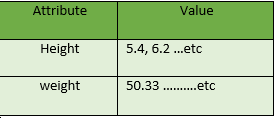My Personal Notes arrow_drop_up
Related Articles